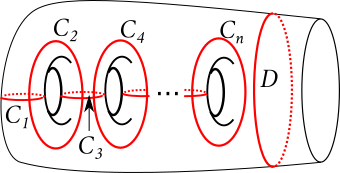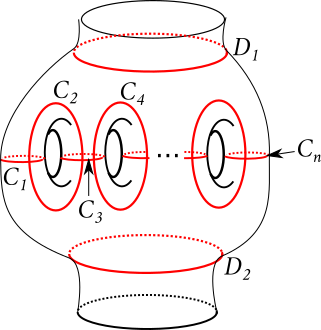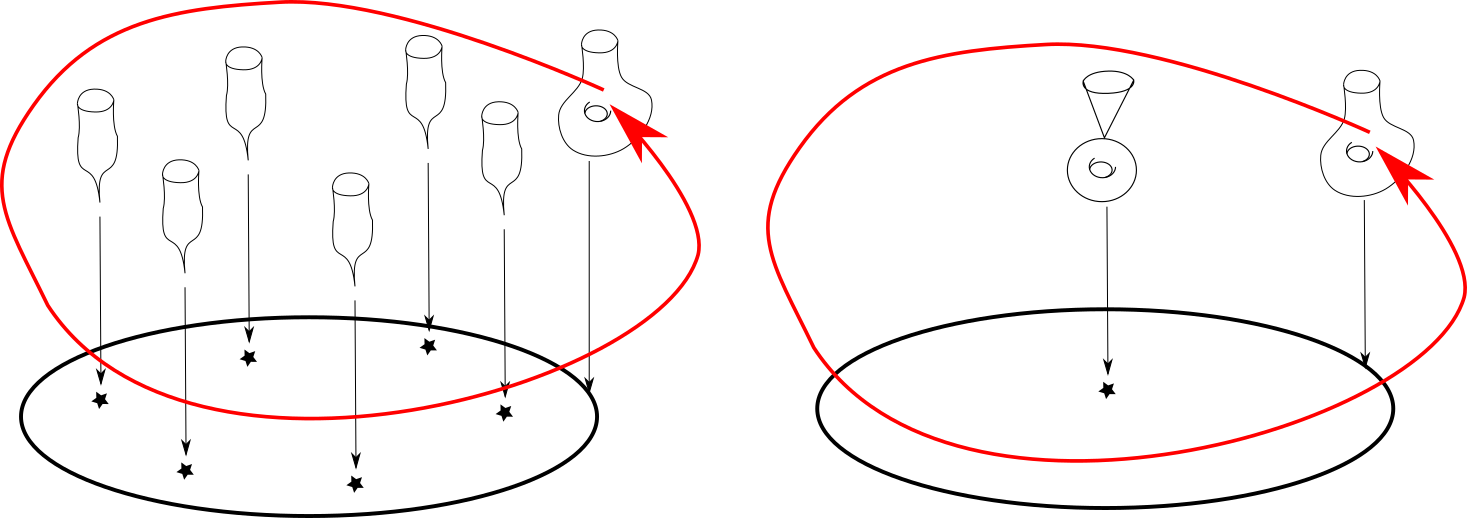# Chain relations

[2019-08-06 Tue]

There is a beautiful series of relations in the mapping class groups of punctured surfaces called the chain relations. In this post, I will explain the origin of these relations in algebraic geometry.

## The relations

If $$n$$ is even, the $$n$$-chain relation tells us that $(\tau_{C_1}\cdots\tau_{C_n})^{2n+2}=\tau_{D}$ where $$\tau_A$$ denotes the right-handed Dehn twist in the curve $$A$$ and $$C_1,\ldots,C_n,D$$ are as shown below.If $$n$$ is odd, the $$n$$-chain relation tells us that $(\tau_{C_1}\cdots\tau_{C_n})^{n+1}=\tau_{D_1}\tau_{D_2}$ for the curves shown below.I recently realised that there's a nice algebro-geometric proof of these relations, which had formerly been somewhat mysterious to me.

## The proof

We'll focus on the $$n$$-even case first.

### Right hand side

Consider the surface $$X\subset\mathbf{C}^3$$ defined by the equation $$x^2+y^{n+1}+z^{2(n+1)}=0$$. This surface has an isolated singularity at the origin. Let $$f\colon\tilde{X}\to X$$ be the minimal resolution. The exceptional locus is a smooth, irreducible curve of genus $$n/2$$. I will justify this below.

Next, consider the projection to the $$z$$ coordinate $$z\colon X\to\mathbf{C}$$. This is a holomorphic map; its fibres are $$\{x^2+y^{n+1}=-z^{2(n+1)}\}$$ which are smooth copies of the $$A_n$$-Milnor fibre if $$z\neq 0$$. This Milnor fibre is the curve shown in the figure for the $$n$$-chain relation (i.e. a once-punctured curve of genus $$n/2$$). The fibre over $$z=0$$ is the singular curve $$x^2+y^n=0$$. This has a single branch passing through the origin, and its proper transform is a smooth copy of $$\mathbf{C}$$ which hits the exceptional locus at a single point.

The composition $$z\circ f\colon\tilde{X}\to\mathbf{C}$$ is now a holomorphic map whose fibres are:

• the smooth $$A_n$$-Milnor fibre over $$z\neq 0$$,
• a plane with a curve of genus $$n/2$$ attached at the origin over $$z=0$$.
This central fibre is obtained from the smooth fibre by collapsing a boundary-parallel loop, i.e. the simple closed curve $$D$$. The monodromy of this fibration is the Dehn twist around $$D$$, i.e. the right hand side of the $$n$$-chain relation.

### Left hand side

Consider the smoothing $$X_\epsilon=\{x^2+y^n+z^{2n}=\epsilon\}$$ of $$X$$ for $$\epsilon\neq 0$$. Again, projecting $$X_\epsilon$$ $$z$$-coordinate we get a holomorphic fibration $$f_\epsilon\colon X_\epsilon\to\mathbf{C}$$ and the monodromy around the unit circle has not changed (up to isotopy) provided $$\epsilon$$ is small enough, so whatever we get will be isotopic to $$\tau_D$$.

The fibration $$f_\epsilon$$ has $$2(n+1)$$ singular fibres at the points where $$z^{2(n+1)}=1$$. Each of these singular fibres ($$x^2+y^n=0$$, $$z$$ fixed) has a single $$A_n$$-singularity. If we pick a vanishing path $$\gamma$$ in the base which connects $$z=1$$ to $$z=0$$ then the vanishing cycle is precisely the $$A_n$$-chain in the smooth fibre over zero. If we apply the automorphism $$(x,y,z)\mapsto (x,y,\mu z)$$ (where $$\mu^{2(n+1)}=1$$) then we get the same vanishing cycle for the vanishing paths $$\mu^k\gamma$$ (because the vanishing cycle lives in $$z=0$$, which is fixed by the automorphism). The monodromy for this fibration is therefore $$\tau^{2(n+1)}$$ where $$\tau$$ is the monodromy for the $$A_n$$-chain (which, it is easy to check, is the product $$\tau_{C_1}\ldots\tau_{C_n}$$).

This implies the chain relation when $$n$$ is even. The picture below attempts to illustrate the case $$n=2$$. The two red arrows indicate the monodromies (which are the same in each picture). The six singular fibres on the left are obtained by collapsing an $$A_2$$-chain in the smooth fibre.### $$n$$ odd

When $$n$$ is odd, we run the same argument with the variety $$X=\{x^2+y^{n+1}+z^{n+1}=0\}$$ (the exceptional locus of the minimal resolution is now a smooth curve of genus $$(n-1)/2$$ and the normalisation of the fibre $$x^2+y^{n+1}=0$$ has two components, so we get two boundary twists).

### The minimal resolution

I want to justify why the minimal resolution of $$x^2+y^{n+1}+z^{2(n+1)}$$ ($$n$$ even) is what I say it is. Make a weighted blow-up at the origin with weights $$n+1,2,1$$, that is consider the surface $\tilde{X}=\{(x,y,z,[a:b:c])\in \mathbf{C}^3\times\mathbf{P}(n+1,2,1)\ :\ x^2+y^{n+1}+z^{2(n+1)}=0,\ \mbox{Plucker}\}$ where Plücker stands for the three relations $$xb=ay,xc=az,yc=bz$$ which become $$[a:b:c]=[x:y:z]$$ if $$(x,y,z)\neq(0,0,0)$$. Here $$\mathbf{P}(n+1,2,1)$$ is the weighted projective space $$\operatorname{Proj}(\mathbf{C}[a,b,c])$$ where $$a,b,c$$ are given degrees $$n+1,2,1$$ respectively. The projection $$f\colon\tilde{X}\to X$$ gives the minimal resolution of $$X$$ and the exceptional locus of $$f$$ is the curve $$\{x^2+y^{n+1}+z^{2(n+1)}=0\}\subset\mathbf{P}(n+1,2,1)$$ which is a smooth curve of genus $$n/2$$ (for background on weighted projective spaces, see [Reid], [Iano-Fletcher], [Dolgachev], [Hosgood]; the last two references explain ways to compute the genus of a smooth weighted plane curve [Dolgachev, Section 3.5.2], [Hosgood, Theorem 3.5.7]).

## References

[Dolgachev] Dolgachev, I. Weighted projective varieties, (1982), in Group actions and vector fields (Vancouver, B.C., 1981), Lecture Notes in Math., 956, Berlin: Springer, pp. 34-71 available from Dolgachev's website and from Springer with DOI: https://doi.org/10.1007/BFb0101508.

[Hosgood] Hosgood, T. An introduction to varieties in weighted projective space, (2016) arXiv:1604.02441

[Iano-Fletcher] Working with weighted complete intersections, in Explicit birational geometry of 3-folds, A. Corti and M. Reid (Editors) CUP 2000. Available from Reid's website in PostScript format and from CUP with DOI: https://doi.org/10.1017/CBO9780511758942.005

[Reid] Reid, M. Graded rings and varieties in weighted projective space. (2002) available from Reid's website.

Comments, corrections and contributions are very welcome; please drop me an email at j.d.evans at lancaster.ac.uk if you have something to share.

CC-BY-SA 4.0 Jonny Evans.